# SUM Function

Sums up cells that are supplied as multiple arguments

## What is the SUM Function?

The SUM function is categorized under Excel Math and Trigonometry functions. The function will sum up cells that are supplied as multiple arguments. It is the most popular and widely used function in Excel.

SUM helps users perform a quick summation of specified cells in MS Excel. For example, we are given the cost of 100 items bought for an event. We can use the function to find out the total cost of the event.

### Formula

=SUM(number1, [number2], [number3]……)

The SUM function uses the following arguments:

1. Number1 (required argument) – This is the first item that we wish to sum.
2. Number2 (required argument) – The second item that we wish to sum.
3. Number3 (optional argument) – This is the third item that we wish to sum.

The function sums values supplied as arguments (up to 255 arguments). Arguments can be supplied as numbers, cell references, ranges, arrays, constants, and the results of other formulas or functions.

### How to use the SUM Function in Excel?

To understand the uses of the SUM function, let’s consider a few examples:

#### Example 1

Suppose we are given the following data: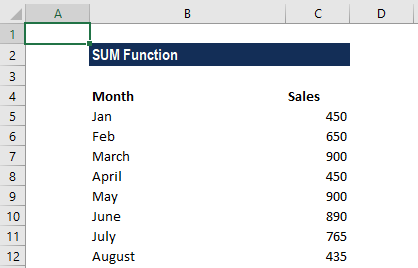We wish to find out the total sales for the first six months. The formula to be used is: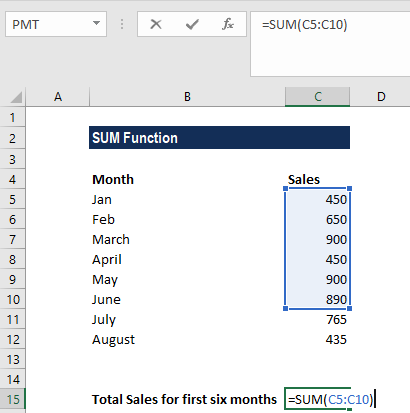We get the result below: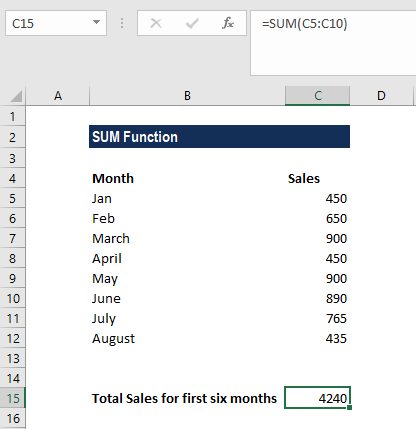#### Example 2

Generally, the SUM function is used as part of bigger formulas used in complex calculations. Suppose we are given the following data: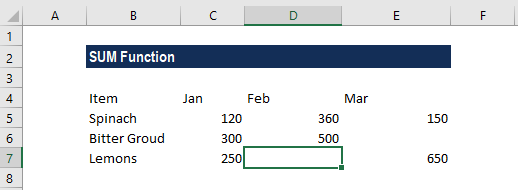As seen above, there is missing information in the data. In such a case, we can use the SUM function along with the IF function to show a warning message. The formula to be used is: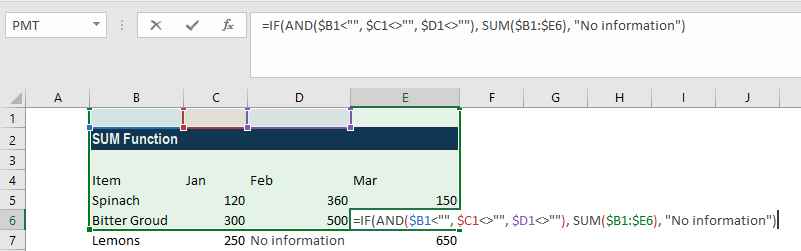We get the result below: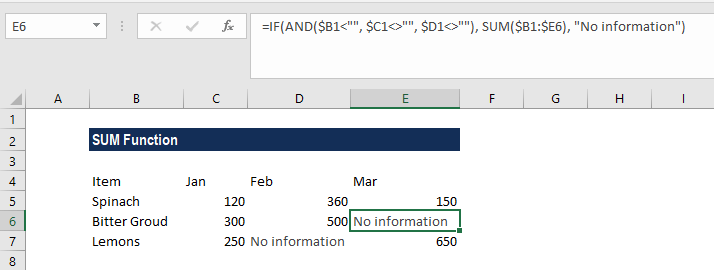### Things to remember about the SUM Function

1. #VALUE! error – Occurs when the criteria provided is a text string that is more than 255 characters long.
2. The easiest way to apply the function is to simply select a cell next to the numbers that we wish to add and click on AutoSum on the Home tab. We can then press the Enter key and the SUM formula is automatically inserted.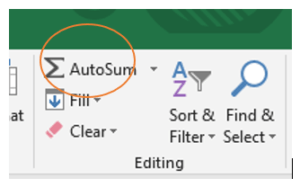We can also find AutoSum in the Formulas tab: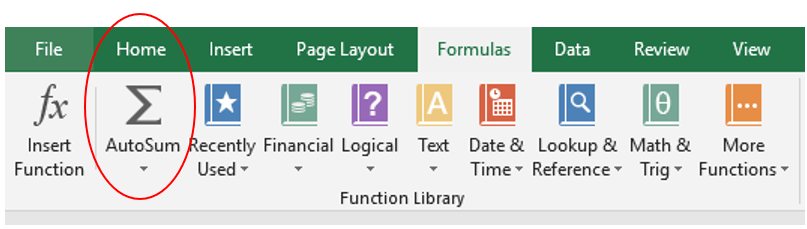The AutoSum feature not only enters a SUM formula but also selects the most likely range of cells that we wish to add up.

Thanks for reading CFI’s guide to the Excel SUM function. By taking the time to learn and master these Excel functions, you’ll significantly speed up your financial analysis. To learn more, check out these additional CFI resources:

• Excel Functions for Finance